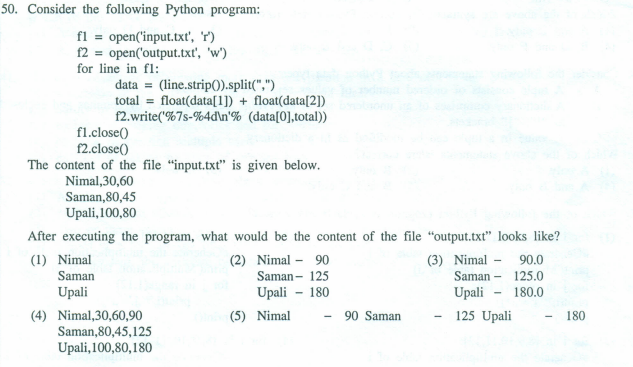# Python 2012 mcqCan anyone explain the 6th line of this code. [f2.write(’%7s-%4d\n’% (data[O],total)]
And what should the answer be?

1 Like

It’s (6th line) formats the text.

• %s means its a string

• %7s means its a string with at least 7 characters

• %d means its a decimal number (integer)

• %2d means its a decimal number with at least 2 characters (digits)

% (data[O],total) <— This part mentions what are the data that put into the %7s and %2d

I have some problems.‘Nimal’ has only 5 characters. If it must be at least 7 characters, can we use that format (% 7s) to show it? Also, the total is only 2 digits. So can we use the % 4d format?

1 Like

If the number of characters is less than the formatting count the rest will filled by spaces.

x="nimal"
y=201
print("%s - %d"%(x,y))

x="nimal"
y=201
print("%8s - %8d"%(x,y))

Try these codes, and check the outputs.
Nimal has only 5 characters. But formatted as 8 characters. the rest fill with spaces as " nimal".

okay. I got it. Thank you so much.1 Like

It’s okay! welcome2 Likes